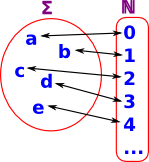# Maths - Number Theory

## Concepts

### Enumerable Sets

 A set Σ is enumerable iff its members can be listed (possibly infinite list) off in some numerical order with every member appearing on the list (allowing repetitions).### Effectively Enumerable

A set Σ is effectively enumerable iff either Σ is empty or there is an effectively computable function that computes it.

theorem

The set W of all effectively enumerable sets of natural numbers is itself enumerable.

theorem

Some sets of numbers are not effectively enumerable and hence not effectively decidable.

#### theorem

There is an effectively enumerable set of numbers K such that its complement

 K
is not effectively enumerable.

theorem

Some effectively enumerable sets of numbers are not decidable.

### Effectively Computable

A one-place total function f: Δ->Γ is effectively computable iff there is an algorithm which can be used to calculate, in a finite number of steps, the value of the function for any given input from the domain Δ.

### Characteristic Function

The characteristic function of the numerical property P is the one-place function
cP: N->Ω such that if n is P, cP(n)=true else cP(n)=false.

(see subobject classifier in category theory/topos).

The characteristic function of the two-place numerical relation R is the two-place function cR: (N,N)->Ω such that if m is R to n,then cR: (m,n)=true else cR: (m,n)=false.

### Effectively Decidable

A property/relation is effectively decidable iff there is an algorithmic procedure that a suitable programmed computer could use to decide, in finite number of steps, whether the property/relation applies to any appropriate given items(s).

or

A numerical property/relation is effectively decidable iff its characteristic function is effectively computable.

### Numerical Domain

The numerical domain of an algorithm Π is the set of natural numbers n such that, when the algorithm Π is applied to the number n as input, then the run of the algorithm will eventually terminate and deliver some number as output.

#### theorem

W is an effectively enumerable set of numbers iff it is the numerical domain of some algorithm Π.